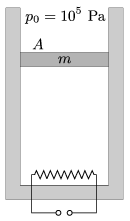Mathematical and Physical Journal
for High Schools
Issued by the MATFUND Foundation
 Already signed up? New to KöMaL?

#Problem P. 4479. (November 2012)

P. 4479. An easily moveable, insulated piston, of cross section A=2 dm2 and of mass m=5 kg, confines some air in a vertical thermally insulated cylinder. The air is heated by a heating element of power 200 W.a) What is the speed of the piston?

b) By what amount does the internal energy of the air change in 2 s?

(5 pont)

Deadline expired on December 10, 2012.

Sorry, the solution is available only in Hungarian. Google translation

Megoldásvázlat. $\displaystyle a)$ $\displaystyle v=2{,}8$ cm/s.

$\displaystyle b)$ 286 J. Ez a fűtőszál által leadott energia 5/7-e, a többi a légkör ,,megemelésére" fordítódik.

### Statistics:

 130 students sent a solution. 5 points: 73 students. 4 points: 14 students. 3 points: 11 students. 2 points: 7 students. 1 point: 20 students. 0 point: 3 students. Unfair, not evaluated: 2 solutionss.

Problems in Physics of KöMaL, November 2012